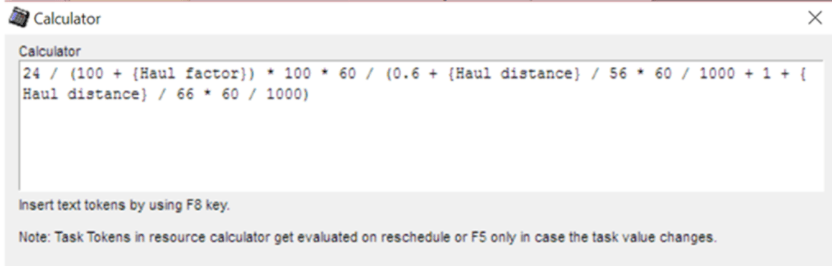# TILOS New Release 9.1 Makes Mass Haulage Even Easier.

This is part one of a 5 part series, where we cover what’s new in TILOS 9.1

# TILOS New Release 9.1 incorporates enhanced Mass Haulage functionality to help project planning and scheduling professionals more effectively execute projects.

Linear infrastructure projects like road and rail projects typically budget anywhere from 30-80% of the entire project budget on earthworks.

Imagine what automated resource calculation could do to keep your project on time and on budget…

## TILOS New Release 9.1 automates mass haulage calculations, taking into account:

The total volume of earth to be moved

How far away it needs to be moved

The total capacity of each excavator

The driving speed and time of each driving resource, both loaded and empty

The data output from TILOS indicates the number of driving resources needed, for how
long, where along the ROW and the associated cost.

Request A Live Demo & Trial Version of TILOS.

### TILOS New Release: 9.1 What’s Different:

The formula for Calculating the Input Value now uses numeric task tokens.  For more information, see the section Dialog – Calculator in Help.

An Example formula is shown below.  Similar formulas can be created.The Formula above is based on workload capacity * cycles per hour.

/ means divided by  and  * means multiply by

The Workload Capacity = 24m3 x 100/115 where the truck volume is 24 and the Haul Factor = 15%  (The material bulks up by 15% in Haulage from its native in ground state)

Cycles per Hour = 60 / Cycle Time (where 60 = minutes in an hour)

Cycle Time (mins) = Load Time + Haul Time (Loaded) + Dump Time + Haul Time (Unloaded)

Haul Time = Distance divided by speed

Outbound and Inbound Haul Time in minutes is computed as follows:

Haul Distance / ((Speed * (1000/60)) and is written (Haul Distance / Speed(kmh) * 60 / 1000)

0.6 = Dump time in minutes

Driving speeds: 25 = Speed Loaded    35 = Speed Empty

Note:

60 / 1000 = conversion from km/h to m/min

Request A Live Demo & Trial Version of TILOS.